Artificial Physics for Artificial Chemistries

(1) artificial chemistries
(2) chemlambda
(3) information content of the deviation from hamiltonian evolution
(4) chemlambda + physics

[Dittrich, P., Ziegler, J., Banzhaf, W. (2001)] - an artificial chemistry is a triple (S,R,A):
S - a class of molecule types for example A, B
R - a class of chemical reactions for exampleA - an algorithm for the application of the chemical reactions to a multiset of molecules, for example random application of a reaction to a random selection of molecules of the right type[K. Tomita, H. Kurokawa, S. Murata (2009)] - a graph-rewrite automaton is a triple (S,R,A):
S - a class of graphs for example trivalent graphs with colored nodes
R - a class of graph-rewrites for example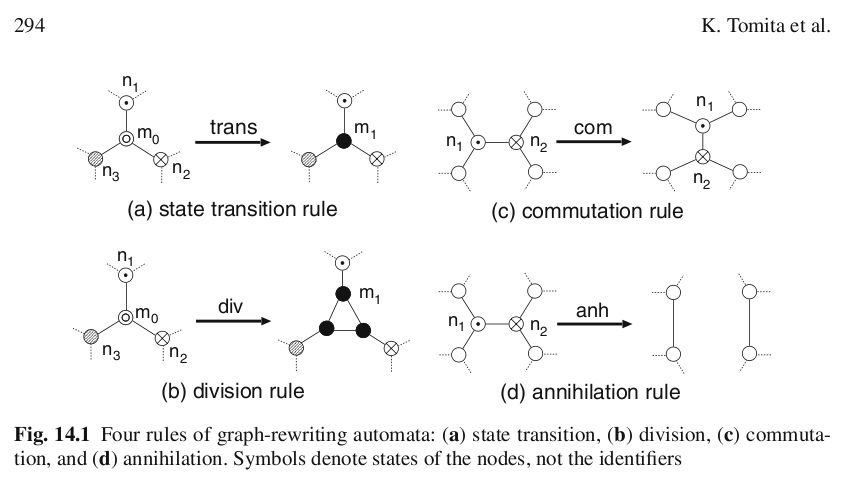A - an algorithm for the application of the graph-rewrites to one graph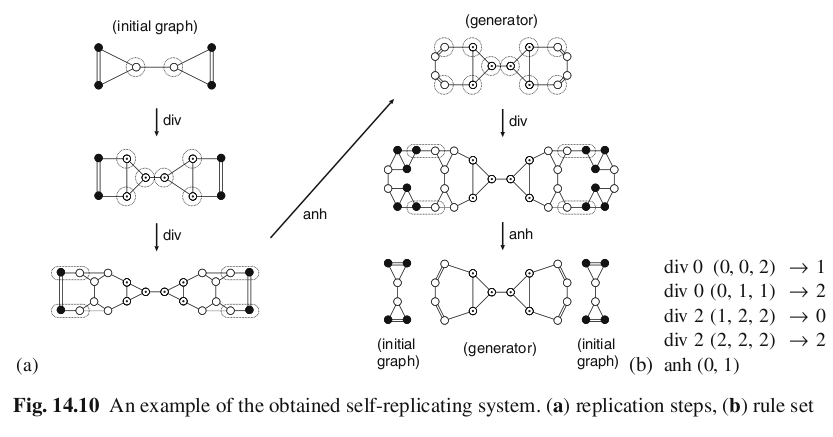an asynchronous graph-rewrite automaton is a triple (S,R,A):
S - a class of "molecules" (i.e. of graphs)
R - a class of "chemical reactions" (i.e. graph-rewrite rules)
A - an algorithm for the application of graph-rewrites to one graph, which is random and local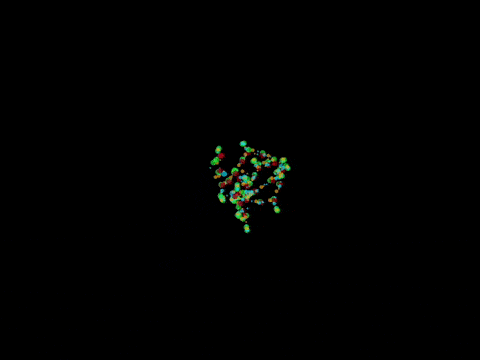λ calculus [A. Church (1936)] string rewrite system
• variable: x, y, z, ...

• term: variable   |   AB where A, B terms   |   λx.A where x var, A term

• β-reduction: (λx.A)B → A[x=B]
[C.P. Wadsworth (1971)], [J. Lamping (1990)] graph rewrite system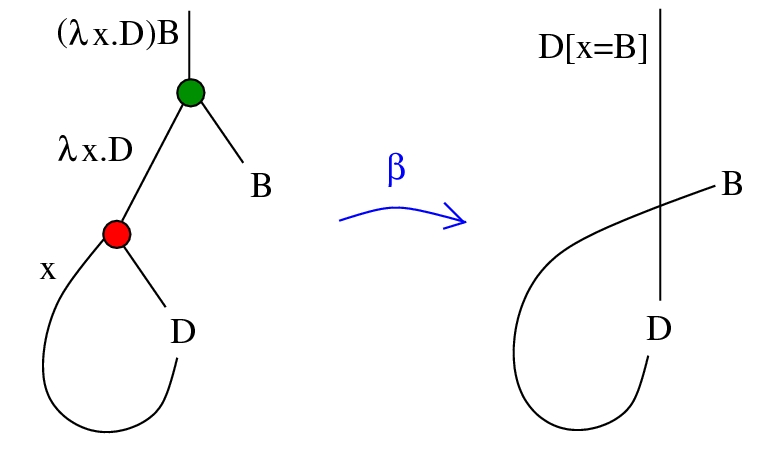[W. Fontana, L. W. Buss (1994)] - ALCHEMY = ALgorithmic Chemistry:
S - a class of λ- terms in normal form
R - a class of reductions

A + B → A + B + normal form of AB

A - algorithm of random application of a reaction to a random selection of terms
[G. Berry, G. Boudol (1992)] - CHAM = Chemical Abstract Machine: a programming language which uses molecules, solutions and transformation rules

[Y. Lafont (1997)] Interaction combinators
S - a class of trivalent fatgraphs
R - a class of graph rewrite rules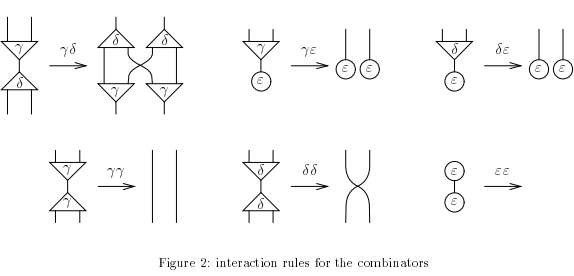A - any reasonable algorithm will do, because the graph-rewrites are strong confluent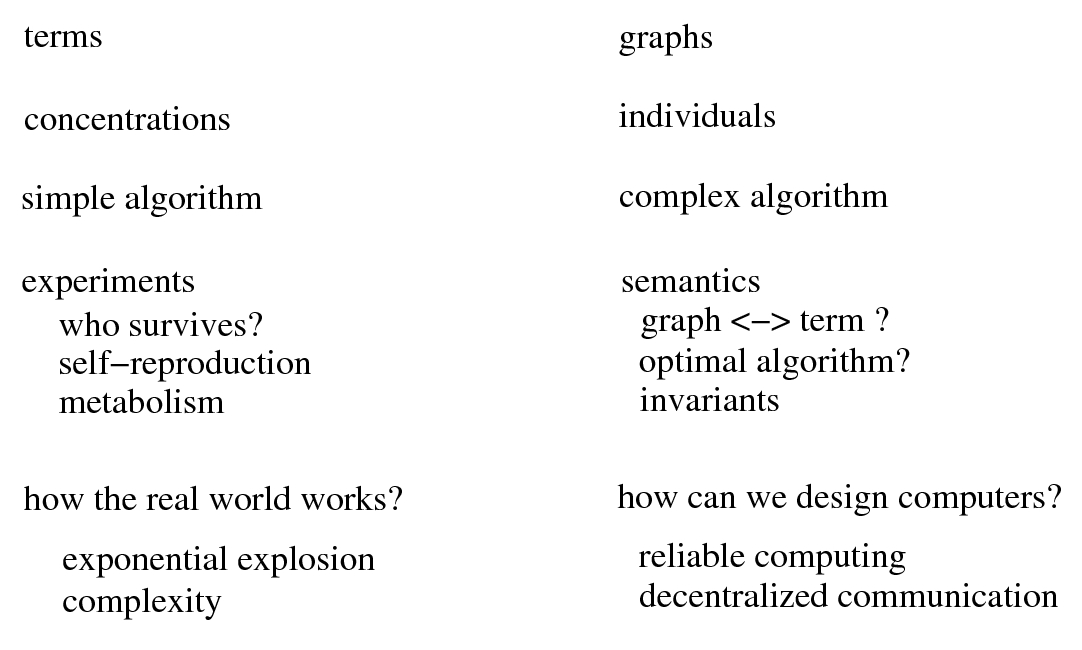The Matrix: what does it mean?

"still he'd see the matrix in his sleep, bright lattices of logic unfolding across that colorless void"

(William Gibson, Neuromancer)

versusTuring machines and λ-terms

[repository] [v2] Chemlambda:
S - a class of trivalent fatgraphsChemlambda:
R - two main families of graph rewrite rulesbeta rewritea DIST rewrite

Chemlambda:
R - a COMB graph rewrite cycle to eliminate the Arrow elements and loops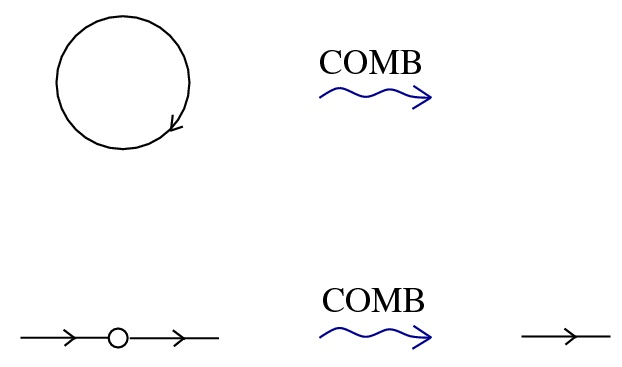COMB cycle

Chemlambda:
A - an algorithm for the application of graph-rewrites to one graph, which is random and local

• randomly pick an edge

• if the edge is part of a pattern for a graph-rewrite then flip a coin to perform the rewrite

• do as many COMB rewrites as possible

• (record the states and the transitions of the graph for further examination)

• repeat until (no more rewrite possible OR too many nodes OR too many rewrites)

• embed the record into a force graph simulation.
There is a deterministic algorithm which uses a list of priorities of rewrites (because they are not strongly confluent)Dodecahedron multiplicationtube pattern duplication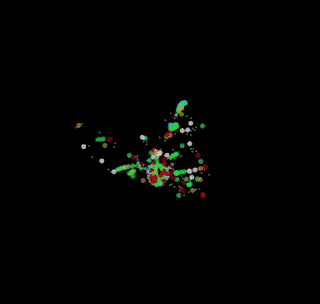tube pattern with Turing Machines, builts itselfthe factorial of 5: (a,b) to (a+1, ab)

Properties:
Turing universal: we can represent λ-terms and rewrites, or TM.
[Ackermann(2,2) simulation]     [Demos]    [Molecular computers (2015)]     arXiv:1811.04960
gives many interesting graphs modified outside λ-calculus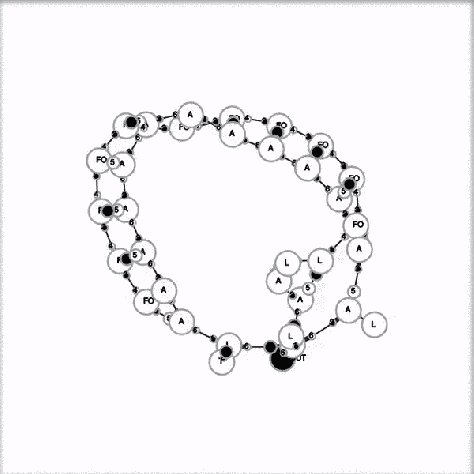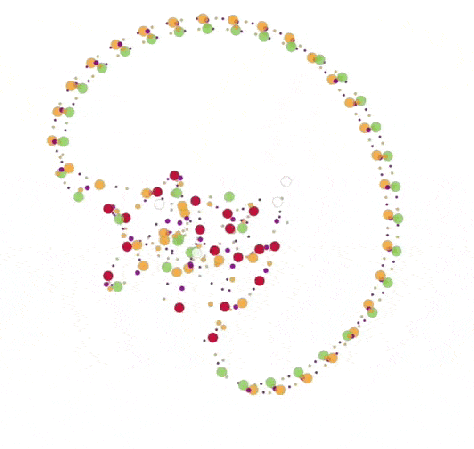artificial life: a chemlambda quine is a graph deterministically periodic, evolving under the random algorithm.
[Library of molecules]     [Collection of simulations]

The information content of the deviation from hamiltonian evolution, an extension of Hamiltonian mechanics. [Buliga (2009)]   [(2018)]   [(2019)]   [Buliga, de Saxce (2017)]
aka "Hamiltonian inclusions with convex dissipation" or "symplectic Brezis-Ekeland-Nayroles principle"
define the deviation from hamiltonian evolution vector ηimpose that the evolution minimizes the information content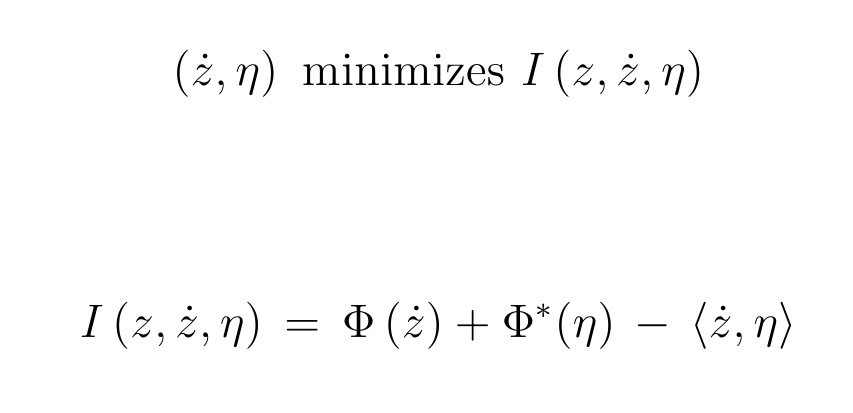allows dissipation (a generalized Liouville theorem)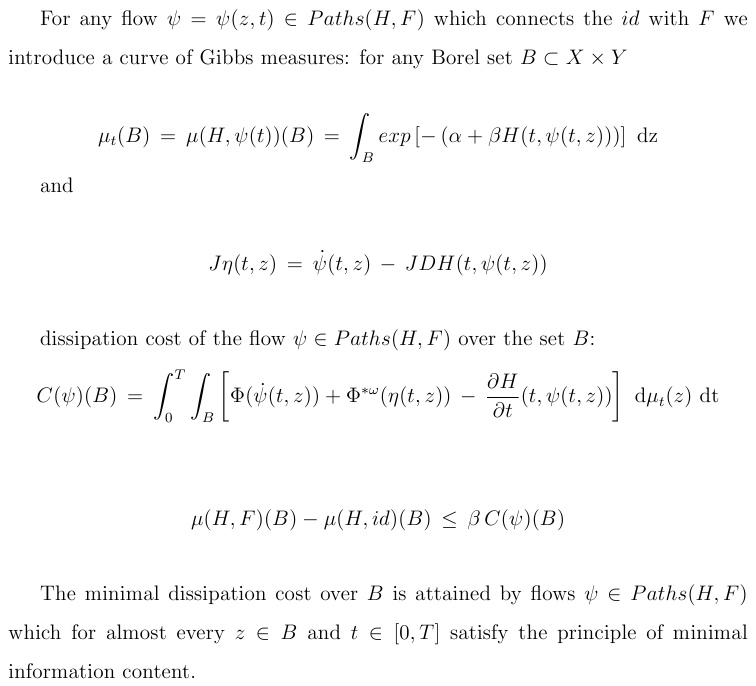applies to: viscosity, plasticity, damage, fracture
also to unilateral contact (and friction)
[E. Fredkin, T. Toffoli (1982)] billiard ball computerLet M be the space (billiard), then the information content function is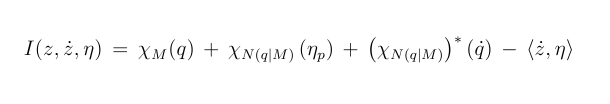stochastic versionchemlambda+physics
A version of chemlambda is a billiard ball computer (with the physics as in the principle of minimal information content), with some of the state variables over a pair of permutohedrachemlambda+physics
anatomy of nodes and edges:chemlambda+physics
initial graph:chemlambda+physics
a rewrite is a pair of permutations:chemlambda+physics
we modify the hamiltonian of the force graph by using the state of the graph
we modify the information content and we use the stochastic version
... and it works (announcement).
CONCLUSION: is a game with a pair of permutohedron dices.

Continue to my homepage